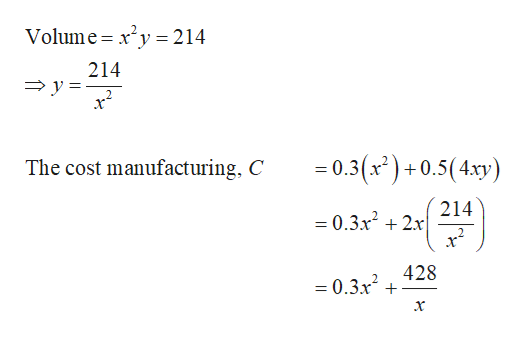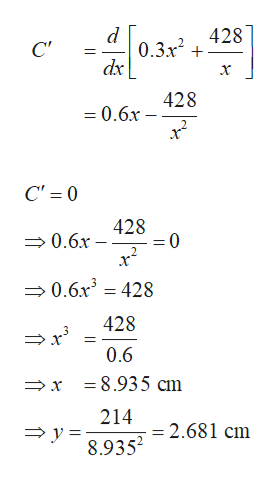# A company plans to manufacture a rectangular container with a square base, an open top, and a volume of 214 cm3. The cost of the material for the base is 0.3 cents per square centimeter, and the cost of the material for the sides is 0.5 cents per square centimeter. Determine the dimensions of the container that will minimize the cost of manufacturing it. What is the minimum cost?

Question
15 views

A company plans to manufacture a rectangular container with a square base, an open top, and a volume of 214 cm3. The cost of the material for the base is 0.3 cents per square centimeter, and the cost of the material for the sides is 0.5 cents per square centimeter. Determine the dimensions of the container that will minimize the cost of manufacturing it. What is the minimum cost?

check_circle

Step 1

Let the side of the square base be x and the height and breadth of the side rectangles be y and x respectively. Given that the volume is 214. Form an equation using these facts as follows.help_outlineImage TranscriptioncloseVolume x2y214 214 y 0.3(x) +0.5(4xy) The cost manufacturing, C 214 =0.3x22x 428 = 0.3x fullscreen
Step 2

Obtain the dimension that minimizes the cost by computing the first der...help_outlineImage Transcriptionclose428 d 0.3x2 dx С" х 428 = 0.6x C' = 0 428 0.6x 0.6x3 =428 428 0.6 =8.935 cm x 214 =2.681 cm y 8.9352 fullscreen

### Want to see the full answer?

See Solution

#### Want to see this answer and more?

Solutions are written by subject experts who are available 24/7. Questions are typically answered within 1 hour.*

See Solution
*Response times may vary by subject and question.
Tagged in

### Calculus